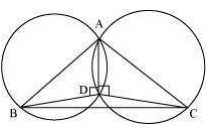Guru

# If circles are drawn taking two sides of a triangle as diameters, prove that the point of intersection of these circles lie on the third side. Q.10

• 0

How i find the best tricky way for solving the question of class 9th of Circles chapter of class 9th give me the best and easy method for this question no.10  of exercise 10.5 If circles are drawn taking two sides of a triangle as diameters, prove that the point of intersection of these circles lie on the third side.

Share

1. First draw a triangle ABC and then two circles having diameter as AB and AC respectively.

We will have to now prove that D lies on BC and BDC is a straight line.Proof:

We know that angle in the semi-circle are equal